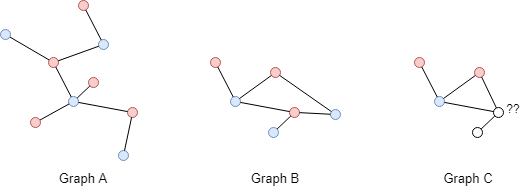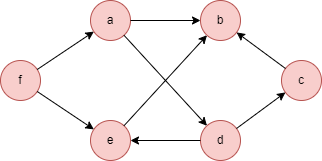# Change Nickname

Current Nickname:

Certifications

Certificate Issued at Valid until Serial No. File
Serial No. Valid until

Not having one? Apply now! >>>

Invoice

ProductName CreateTime ID Price File
ProductName CreateTime ID Price File

# Bipartite Graph

## Overview

Bipartite algorithm judges if the current graph is a bipartite graph and returns the result. The concept of bipartite graph is widely used in scenarios such as resources allocation and grouping optimization.

## Basic Concept

### Bipartite Graph

Bipartite graph is also known as bigragh. If divides all nodes in a graph into sets A and B, the two endpoints i and j of each edge in the graph belong to each set, i.e. i ∈ A and j ∈ B, or i ∈ B and j ∈ A, then the graph is called a bipartite graph.As shown in the above graph, after dividing nodes in the graph into two groups of 'yellow, red, purple' and 'blue, grey, green', there is no edge in the groups, but all edges exist between groups, thus we say that the original graph is a bipartite graph.

Drawing an open curve to divide nodes in a graph into two parts, meanwhile assuring that all edges in the graph intersect the curve only once, the following can be achieved if the graph is a bipartite graph: when the curve is closed, each edge in the graph still intersects the curve only once.Observe the example above, the curve in Graph A meets the requirement of bipartite graph, so Graph A is a bipartite graph; the curve in Graph B intersects one edge twice inevitably, so Graph B is not a bipartite graph.

A cycle with even nodes is called an Even Cycle, a cycle with odd nodes is called an Odd Cycle. Reasoning from the example above: Cycles in bipartite graph must all be even cycles. Because when the curve crosses the edges of an even cycle in turn, the start and end points of the curve are on the same side of the cycle (inside or outside); however, odd cycle causes the start and end points of the curve on different sides of the cycle, so the curve has to intersect with one edge twice to close itself.

### Coloring Method

Coloring is one of the fundamental methods to determine bipartite graph. Since bipartite graph requires that the nodes to be divided into two sets, and no edge may connect two nodes that are in the same set, so all nodes in the graph can be colored in two different colors. The principle of coloring is that the adjacent nodes are colored with different colors. If it is found that a same color is used for adjacent nodes, or different colors appear in the multiple neighbors of a node, then it can be concluded that the graph is not a bipartite graph.In the example above, Graph A and B are colored successfully, but Graph C fails. The coloring method proves the previous reasoning of 'Even Cycle' from another perspective: When coloring nodes along the cycle, even cycle ensures that the nodes are alternately colored in different colors, while the first and last nodes in odd cycle have to use the same color.

## Special Case

### Lonely Node, Disconnected Graph

Introducing pure lonely node (without self-loop edge) into graph does not affect the bipartition of the original graph.

Bipartition is examined for each connected component in disconnected graph, only when all the connected components are bipartite graph, the original graph is bipartite graph.

### Self-loop Edge

Two endpoints of self-loop edge are the same node, thus graph that involves self-loop edge does not meet the requirement of bipartite graph.

### Directed Edge

For directed edges, Bipartite algorithm ignores the direction of edges but calculates them as undirected edges.

## Results and Statistics

Take the graph below as an example, run the Bipartite algorithm:Algorithm results: N/A

Algorithm statistics: The judgement result `bipartite_result`, 1 means the graph is bipartite graph

bipartite_result
1

## Command and Configuration

• Command: `algo(bipartite)`
• There is no configuration for the parameter `params()`

## Algorithm Execution

#### 1. File Writeback

Not supported by this algorithm.

#### 2. Property Writeback

Not supported by this algorithm.

#### 3. Statistics Writeback

Not supported by this algorithm.

### Direct Return

Alias Ordinal
Type
Description
Column Name
0 KV Result of the bipartite judgement, 0 means false, 1 means true `bipartite_result`

Example: Examine if the current graph is bipartite graph, define algorithm statistics as alias named result, and return the statistics

``````algo(bipartite).params() as result
return result
``````

### Streaming Return

Not supported by this algorithm.

### Real-time Statistics

Alias Ordinal
Type
Description
Column Name
0 KV Result of the bipartite judgement, 0 means false, 1 means true `bipartite_result`

Example: Examine if the current graph is bipartite graph, define algorithm statistics as alias named result, and return the statistics

``````algo(bipartite).params().stats() as result
return result
``````